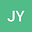•n this paper, we focus on the energy conservation for the weak solutions of inhomogeneous Navier-Stokes equations. It is proved that if the function of density belongs to $L^{\infty}(0,T;L^{\infty}(\mathbb{T}^N))\cap L^p(0,T;W^{1,p}(\mathbb{T}^N))$, and the function of velocity belongs to $L^s(0,T;L^r(\mathbb{T}^N))$ with $\f2s+\f2r=1$, then the energy equality holds. This result can be seen as a inhomogeneous version for Shinbrot’s criterion.